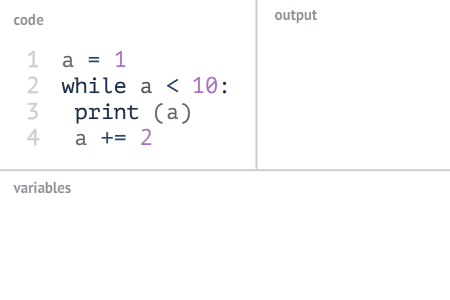Python3.8.5
Vim7.4.629

# 二、变量

Python 3 中的变量命名有一定要求

1. 必须要字母及下划线开始
2. 其他符号可以是数字、字母或下划线
3. 命名区分大小写，且不能使用关键字

# 三、数据类型

## 3.1 数字

1. int 整数类型
2. float 浮点数
3. bool 布尔型
4. complex 复数

#! /usr/bin/env python3

a = 100
b = 12.2
c = True
d = 4+3j

print(type(a),a)
print(type(b),b)
print(type(c),c)
print(type(d),d)

[root@localhost 2]# python3 3.1.py
<class 'int'> 100
<class 'float'> 12.2
<class 'bool'> True
<class 'complex'> (4+3j)


## 3.2 字符串

Python中的字符串用单引号 ' 或双引号 " 括起来，同时使用反斜杠 \ 转义特殊字符。

#! /usr/bin/env python3

a = "100"
b = 'hello'
c = "word"
d = '"hell，word"'
e = "\"hello\",\"word\""

print(type(a),a)
print(type(b),b)
print(type(c),c)
print(type(d),d)
print(type(e),e)

[root@localhost 2]# python3 3.2.py
<class 'str'> 100
<class 'str'> hello
<class 'str'> word
<class 'str'> "hell，word"
<class 'str'> "hello","word"


## 3.3 列表

#!/usr/bin/env python3

a = [ 'abcd', 786 , 2.23, 'awe', 70.2 ,[3,2,1]]

print(type(a))

[root@localhost 2]# python3 3.3.py
<class 'list'>


## 3.4 元组

#!/usr/bin/env python3

a = ( 'abcd', 786 , 2.23, 'awe', 70.2 ,[3,2,1] )

print(type(a))

[root@localhost 2]# python3 3.4.py
<class 'tuple'>


## 3.5 字典

#!/usr/bin/env python3

a = { 'a':2 , 'b':4 , 'c':'h' , 'd':"p" }

print(type(a))

[root@localhost 2]# python3 3.5.py
<class 'dict'>


# 四、运算

## 4.1 数学运算

#!/usr/bin/env python3

a = 100
b = 100.2

# 加法
e = a + b
# 减法
f = b - a
# 乘法
g = b * a
# 除法
h = b / a
# 整除
i = b // a
# 取余
j = b % a
# 乘方
k = b ** a

print(e)
print(f)
print(g)
print(h)
print(i)
print(j)
print(k)

[root@localhost 2]# python3 4.1.py
200.2
0.20000000000000284
10020.0
1.002
1.0
0.20000000000000284
1.2211588271895953e+200


## 4.2 或非运算

and 表示与运算，只有两个运算值都是 True 才返回 True
or 表示或运算，有一个为 True 则返回 True

#!/usr/bin/env python3

a = True
b = False

c = a and b
d = a or b
e = a and a and b
f = a or b or a
g = a or b and b

print(c)
print(d)
print(e)
print(f)
print(g)

[root@localhost 2]# python3 4.2.py
False
True
False
True
True


## 4.3 字符串运算

#!/usr/bin/env python3

a = "hello,word"

# 取第一个字符
print (a)

# 取第二个字符
print (a)

# 取倒数第一个字符
print (a[-1])

# 取倒数第二个字符
print (a[-2])

# 取前两个字符
print (a[:2])

# 取前三个字符
print (a[:3])

# 取第三个字符后的所有字符
print (a[2:])

# 取第四个字符后的所有字符
print (a[3:])

# 取第二到第四个字符
print (a[1:4])

# 输出字符串两次
print (a * 2)
# 连接字符串
print (a + "TEST")


[root@localhost 2]# python3 4.3.py
h
e
d
r
he
hel
llo,word
lo,word
ell
hello,wordhello,word
hello,wordTEST


# 五、结构控制

## 5.1 选择控制

#!/usr/bin/env python3

a = int(input("Please enter Number: "))

if a > 10:
print('a > 10')
elif a == 10:
print('a == 10')
else:
print('a < 10')

[root@localhost 2]# python3 5.1.py
a > 10


## 5.2 循环控制

Python 中包含两种循环方式，一种是 for，一种是 while

for 循环主要用在依次取出一个列表中的项目，对列表进行遍历处理。下一节中我们将详细讲解列表的数据结构，这里可以简单理解为一组值。

#!/usr/bin/env python3

strlist = ['hello','word','python3']
for i in strlist:
print(i)

[root@localhost 2]# python3 5.2.1.py
hello
word
python3


(图片来自菜鸟教程)#!/usr/bin/env python3

a = 1
while a < 10:
print(a)
a += 2

[root@localhost 2]# python3 5.2.2.py
1
3
5
7
9


break 表示停止当前循环，continue 表示跳过当前循环轮次中后续的代码，去执行下一循环轮次。

#!/usr/bin/env python3

for a in range(10):
if a == 5:
break
print(a)

[root@localhost 2]# python3 5.2.3.py
0
1
2
3
4


#!/usr/bin/env python3

a = 100
while a > 10:
a -= 10
if a == 50:
continue
print(a)

[root@localhost 2]# python3 5.2.4.py
90
80
70
60
40
30
20
10


# 六、异常处理

Python 3 中常见的异常类型:

• NameError 访问一个未定义的变量
• SyntaxError 语法错误，这个严格讲算是程序的错误
• IndexError 对于一个序列，访问的索引超过了序列的范围，可以理解为我的序列里只有三个元素，但要访问第4个
• KeyError 访问一个不存在的字典 Key，Key 如果不存在字典就会抛出这个异常
• ValueError 传入无效的参数
• AttributeError 访问类对象中不存在的属性

## 6.1 try/except

(图片来自菜鸟教程)#!/usr/bin/env python3

try:
x = int(input("请输入一个数字: "))
except ValueError:
print("您输入的不是数字！")

[root@localhost 2]# python3 6.1.py



## 6.2 try/except/finally

finally 关键字是用来进行清理工作，经常和 except 一起使用，即无论是正常还是异常，这段代码都会执行。

(图片来自菜鸟教程)#!/usr/bin/env python3

try:
x = int(input("请输入一个数字: "))
except ValueError:
print("您输入的不是数字！")
finally:
print("程序结束")

[root@localhost 2]# python3 6.2.py

[root@localhost 2]# python3 6.2.py



# 七、引入模块

from urllib2 import urlopen11-021882
04-04741
03-205147
06-0163
11-293893